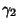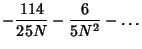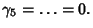## Spearman Rank Correlation Coefficient

A nonparametric (distribution-free) rank statistic proposed by Spearman in 1904 as a measure of the strength of the associations between two variables (Lehmann and D'Abrera 1998). The Spearman rank correlation coefficient can be used to give an R-Estimate.

The Spearman rank correlation coefficient is defined by(1)

whereis the difference in Rank of corresponding variables, and is an approximation to the exact Correlation Coefficient(2)

computed from the original data. Because it uses ranks, the Spearman rank correlation coefficient is much each to compute.

The Variance, Kurtosis, and higher order Moments are(3)(4)(5)

Student was the first to obtain the Variance.